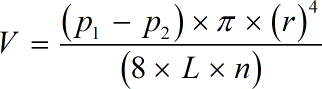Request a Tool

Poiseuilles Equation Calculator

Viscosity refers to the measure of a fluid's resistance to flow. Kinematic Viscosity is defined as the fluid's absolute that is divided by its density.

Velocity
0

Formula• p1 = Initial Pressure
• p2 = Final Pressure
• r = Internal Radius of the Tube
• n = Absolute Viscosity
• L = Total Length of the Tube
• V = Volume per Second

Defination / Uses

In fluid dynamics computations, the Poiseuille equation is a simple formula. The laminar flow in a cylindrical container is described by this equation.

How to use poiseuilles equation calcultor?

There is only single basic step.

• Add all required values in their corresponding fields.